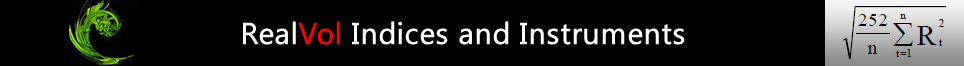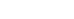# Types of RealVol Indices

### RealVol Daily Indices

All RealVol Daily Indices come in six time frames: 1-day, 1-week (5 trading days), 1-month (21 trading days), 1-quarter (63 trading days), 1-half year (126 days), and 1-year (252 trading days). The six time frames correspond to the time horizons of most traders (short-term, medium-term, and long-term, respectively). For periods longer than one year, realized volatility deviates little from its very longterm average so is generally estimated to be a constant.

### VOL Indices

The realized volatility indices (VOL) measure the inter-day (close-to-close) realized volatility of an underlying using the RealVol Daily and Real-Time Formula. As discussed, there are six time frames for all indices including VOL. The special flagship VOL Index is based on the 1-month time frame only. The reason for flagship status is that the 1-month version is the only one used for tradable instruments.

### VOV Indices

Realized volatility of realized volatility indices (VOV) measure the realized volatility of VOL using the RealVol Daily Formula a second time on the same data. Trading instruments on realized volatility compels one to discover the risk of those instruments. To do so, one needs to calculate the vol of vol. The VOV indices attempt to quantify such risk and can be especially useful as a guide to options traders of realized volatility instruments.

### DVOL Indices

Overnight/intraday realized volatility indices (DVOL) measure the realized volatility using previous day's close and today's open, high, and low data of an underlying using the RealVol Overnight/Intraday Formula. One can think of DVOL as another measure of 24-hour price risk of an underlying using more data points for greater accuracy.

### VCOR Indices

Correlation indices (VCOR) measure the correlation between the underlying and its VOL. They are calculated by using the RealVol Correlation Formula. Use VCOR for insight into the linear relationship between the underlying and its realized volatility.

### VAR Indices

Realized variance indices (VAR) measure the inter-day realized variance of an underlying using the RealVar Daily Formula. One can think of VAR as another measure of inter-day price risk of an underlying. Variances are easier to sum, average, and combine because the result is linear as opposed to volatility, which is a curve function.

### RVOL Indices

The Rough Vol model (Rough Fractional Stochastic Volatility or “RFSV”) forecasts realized volatility.  According to the model, created by Professor James Gatheral of Baruch College, the log of daily high/low realized volatilities is well approximated by fractional Brownian motion with a Hurst parameter H close to zero.  RealVol uses the Rough Vol model to create the RVOL indices, which forecast the VOL index over six standardized time frames.  Our preliminary research has shown that the Rough Vol model approximates future realized volatility more accurately than the options market (implied volatility) and certain GARCH models.  Both the HARK (see below) and Rough Vol models have similar long-run predictive accuracy, but arrive at their forecasts in quite different manners — often diverging significantly over short periods of time.

### HVOL Indices

HARK (Heterogeneous auto-regressive model cast into a Kalman filter framework) is a forecast of realized volatility created by Professor Fulvio Corsi of the University of Pisa and City University of London.  It is a dynamic extension of the asymmetric (i.e., with leverage effects) HAR model where the parameters are continuously and optimally updated by the Kalman filter according to the statistical properties of an intraday realized volatility input.  This allows flexibility and fast adaptation to the original HAR model.  The HARK model is used to create the HVOL indices, which forecast realized volatility over six standardized time frames.